Next: Proposed solutions to attenuate Up: Noise attenuation by filtering Previous: Introduction

# A short review of inverse problems

In this section I review some basic notions on inversion. The least-squares criterion comes directly from the hypothesis that the PDF of each observable data and each model parameter is gaussian. These assumptions lead to the general discrete inverse problem Tarantola (1987). Finding m is then equivalent to minimizing the quadratic function (or cost/objective function)(14)
where ()' represents the (Hermitian) transpose,is a mapping of the data (unknown of the inverse problem),a seismic (linear) operator,the seismic data,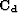and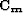the data and model covariance operators anda model given a priori.

The covariance matrixcombines experimental errors and modeling uncertainties. Modeling uncertainties describe the difference between what the operator can predict and what is contained in the data. Thus the covariance matrixis often called the noise covariance matrix Sacchi and Ulrych (1995). It is often assumed that, (1) the variances of the model and of the noise are uniform, (2) the covariance matrices are diagonal, i.e., the model and data components are uncorrelated, and (3) no prior model is known in advance. Given these approximations the objective function becomes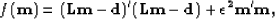(15)
where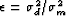is a function of the of the noise and model variances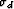and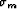. The model perturbationreduces to a damping of the cost function. In practice, this damping is used to compensate for numerical instabilities when the parameters (m) are poorly constrained.

The prior assumptions leading to equation () are often too strong when dealing with seismic data because the variance of the noise/model may be not uniform and the components of the noise/model not independent. For simplicity I rewrite the objective function in equation () in terms of fitting goals'' for m as follows:(16)
where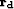is the vector of data residuals and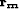is the vector of model residuals. With this notation, it is straightforward to rewrite equation () as(17)
The first equality in equation () stresses the need for Lm to fit the input data. The second equality is often called the regularization or model styling'' term. This term can be useful for imposing a-priori knowledge on the model parameters Clapp et al. (2004); Fomel and Claerbout (2003). When the assumptions leading to equation () are respected, the estimated model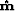that minimizes equation () is the maximum likelihood model Tarantola (1987).

As stressed before, an important assumption made in equation () is that the data and model errorsandare IID. In the situation where coherent noise contaminates the data, these assumptions are violated and the covariance operators cannot be approximated with diagonal operators anymore.

In this Chapter, omitting the model residual vectorin the analysis, I show that a filtering (or weighting) operator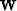can be introduced in equation () such that. This operator can take the form of a prediction-error filter or a projection filter.Next: Proposed solutions to attenuate Up: Noise attenuation by filtering Previous: Introduction
Stanford Exploration Project
5/5/2005